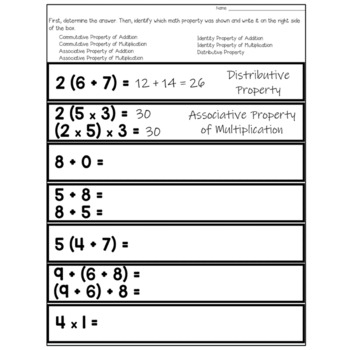digital

# Math Properties Sorting ActivitySubject
Resource Type
Formats

TpT Digital Activity

PDF (2 MB|8 pages)
Standards
\$3.00
TpT Digital Activity
Add notes & annotations through an interactive layer and assign to students via Google Classroom.
\$3.00
TpT Digital Activity
Add notes & annotations through an interactive layer and assign to students via Google Classroom.

### Description

Math Properties Sorting Activity: Your students will complete the equations on 21 strips, and then identify the math property they used from the list below.

- Commutative Property of Multiplication

- Associative Property of Multiplication

- Identity Property of Multiplication

- Distributive Property

Once students are done sorting, they cut out their strips and slide them inside the appropriate pocket. (Directions on how to make the pockets are included.)

This project can be assembled on construction paper and made into a bulletin board or hallway display. The pockets can also be glued into interactive notebooks!

Are you wondering why I chose the images with each property that I did? Check out the PREVIEW!

By Deb Hanson

Total Pages
8 pages
Included
Teaching Duration
45 minutes
Report this Resource to TpT
Reported resources will be reviewed by our team. Report this resource to let us know if this resource violates TpT’s content guidelines.

### Standards

to see state-specific standards (only available in the US).
Apply properties of operations as strategies to multiply and divide. Examples: If 6 × 4 = 24 is known, then 4 × 6 = 24 is also known. (Commutative property of multiplication.) 3 × 5 × 2 can be found by 3 × 5 = 15, then 15 × 2 = 30, or by 5 × 2 = 10, then 3 × 10 = 30. (Associative property of multiplication.) Knowing that 8 × 5 = 40 and 8 × 2 = 16, one can find 8 × 7 as 8 × (5 + 2) = (8 × 5) + (8 × 2) = 40 + 16 = 56. (Distributive property.)